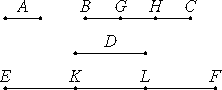# Proposition 15

If a unit measures any number, and another number measures any other number the same number of times, then alternately, the unit measures the third number the same number of times that the second measures the fourth.

Let the unit A measure any number BC, and let another number D measure any other number EF the same number of times.

I say that, alternately also, the unit measures the number D the same number of times that BC measures EF.Since the unit A measures the number BC the same number of times that D measures EF, therefore there are as many numbers equal to D in EF as there are units in BC.

Divide BC into the units in it, BG, GH, and HC, and divide EF into the numbers EK, KL, and LF equal to D. Then the multitude of BG, GH, and HC equals the multitude of EK, KL, and LF.

And, since the units BG, GH, and HC equal one another, and the numbers EK, KL, and LF also equal one another, while the multitude of the units BG, GH, and HC equals the multitude of the numbers EK, KL, and LF, therefore the unit BG is to the number EK as the unit GH is to the number KL, and as the unit HC is to the number LF.

VII.12

Since one of the antecedents is to one of the consequents as the sum of the antecedents is to the sum of the consequents, therefore the unit BG is to the number EK as BC is to EF.

But the unit BG equals the unit A, and the number EK equals the number D. Therefore the unit A is to the number D as BC is to EF.

VII.Def.20

Therefore the unit A measures the number D the same number of times that BC measures EF.

Therefore, if a unit number measures any number, and another number measures any other number the same number of times, then alternately, the unit measures the third number the same number of times that the second measures the fourth.

Q.E.D.

## Guide

Euclid shows that if b = nu, and e = nd, and d = mu, then e = mb. As a single equation,

n(mu) = m(nu)

This proposition expresses the commutativity of multiplication, but it is a commutativity with regard multiple multiples of the the unit u. The next proposition states a commutativity of multiplication of formal numbers.

#### Outline of the proof

Proposition VII.12 said that

if a1 : b1 = a2 : b2 = ... = an : bn,

then each of these ratios also equals the ratio

(a1 + a2 + ... + an) : (b1 + b2 + ... + bn).

Now take all the ai’s to be the unit u, all the bi’s to be d, then conclude

u : d = nu : nd.

But d = mu. Therefore nd = m(nu), as required. Q.E.D.

This proposition is used in the next proposition and a few others in Books VII and IX.